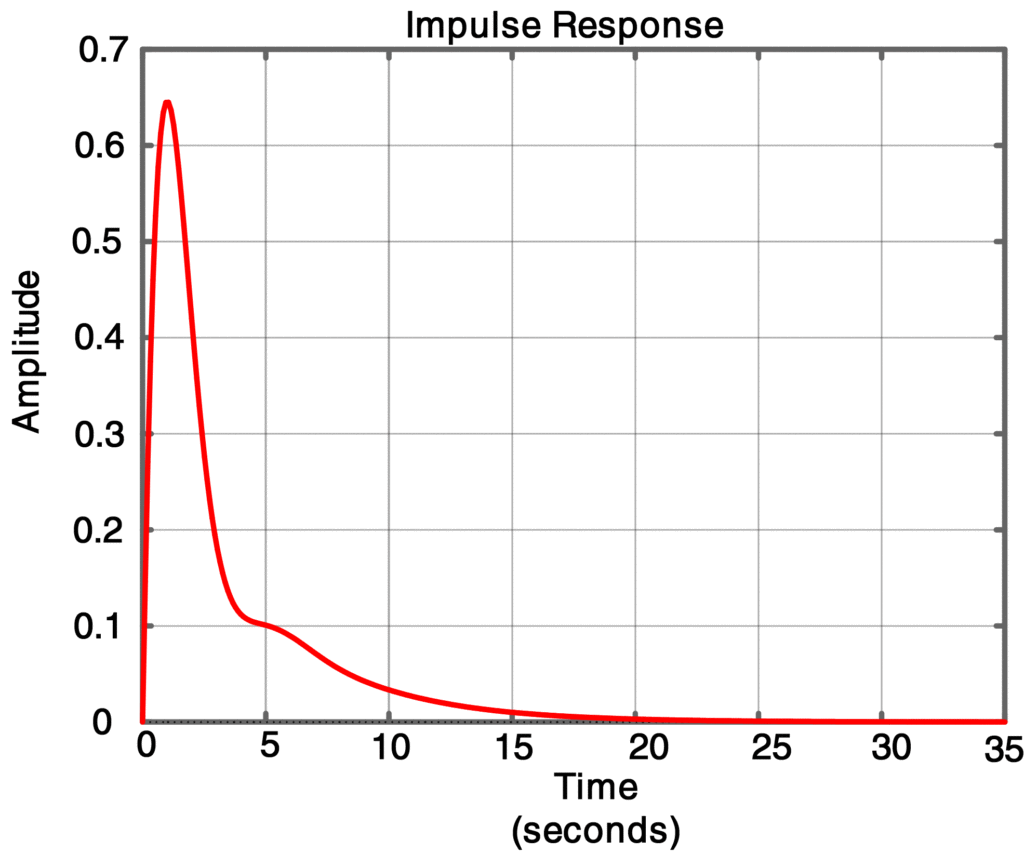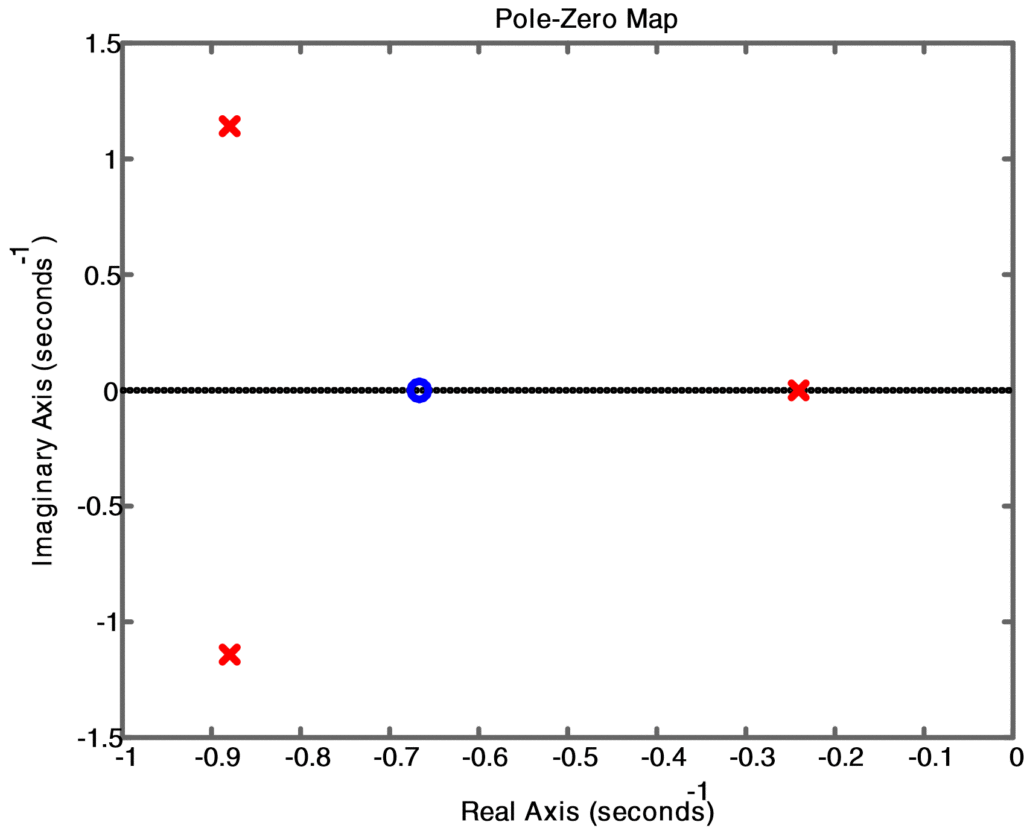Home / Control with Matlab / System Stability Analysis using MATLAB

# System Stability Analysis using MATLAB

Want create site? Find Free WordPress Themes and plugins.

## Poles and Stability

If pi is a pole of G(s), then the natural, or zero-input, the response of G(s) will consist of the mode functions epit if pi is distinct, and tq epit, q = 0, 1,. . ., r – 1, if pi has multiplicity r. Thus the natural response will decay to zero if Re[pi] < 0 for i = 1, . . . , n-that is, if all the poles are in the open left-half of the s-plane, i.e., the left half-plane excluding the imaginary axis. Such a system is said to be asymptotically stable.

If all the poles are in the open left-half plane except for distinct poles on the imaginary axis and at the origin, the natural response will consist of undamped sinusoids or a nonzero constant, and the system is said to be marginally stable.

If some of the poles are in the right-half of the s-plane or on the imaginary axis with multiplicity greater than one, then the natural response will be unbounded and the system is said to be unstable.

## Determine Transfer Function Poles

For G(s) given in TF form, the MATLAB function tf2zp can be used to determine the poles of G(s) and its stability. Alternatively, since we are interested only in the poles, we can apply the polynomial roots function root to the denominator of G(s) to ﬁnd its poles. The following example illustrates the use of roots to determine the system’s stability.

## System Stability using Matlab Example

Find the poles of the transfer function

$G(s)=\frac{1.5s+1}{{{s}^{3}}+2{{s}^{2}}+2.5s+0.5}$

and determine whether the system is stable. Plot the poles and zeros of G(s) in the s-plane. Finally, demonstrate the system stability by simulating the impulse response.

Solution

Deﬁne the row vectors numG = [1.5 1] and denG = [1 2 2.5 0.5] to represent the numerator and denominator of G(s), respectively. Using the command roots (denG), we ﬁnd the poles of G(s) to be at s = -0.2408 and -0.8796 ± j1.1414. Since all the poles have negative real parts, that is, they are all in the open left-half plane, the system is asymptotically stable. The impulse response can be found from the command impulse (numG, denG), and will decay to zero as shown in figure 1. The MATLAB commands in Script 7 will compute the poles and zeros and make the plot as shown in figure 2.

From roots command, we obtained the following result:

 Poles -0.8796 + 1.1414i -0.8796 – 1.1414i -0.2408 + 0.0000i

## Matlab Code to find poles and impulse response


% Script 7: Matlab Code to find poles and impulse response

clear all;close all;clc

numG = [1.5 1]; % Create G(s) as a ratio of numerator &amp;amp;amp;amp; denumerator

denG = [1 2 2.5 0.5];

roots(denG) % find poles of G(s)

pzmap(numG,denG) % plot the poles and zeros

impulse(numG,denG) % simulate impulse response



ResultFig.1: Impulse Response of a Transfer FunctionFig.2: Pole Zero Plot for System Stability

Did you find apk for android? You can find new Free Android Games and apps.